Examples

Chapter 9 Class 12 Differential Equations
Serial order wise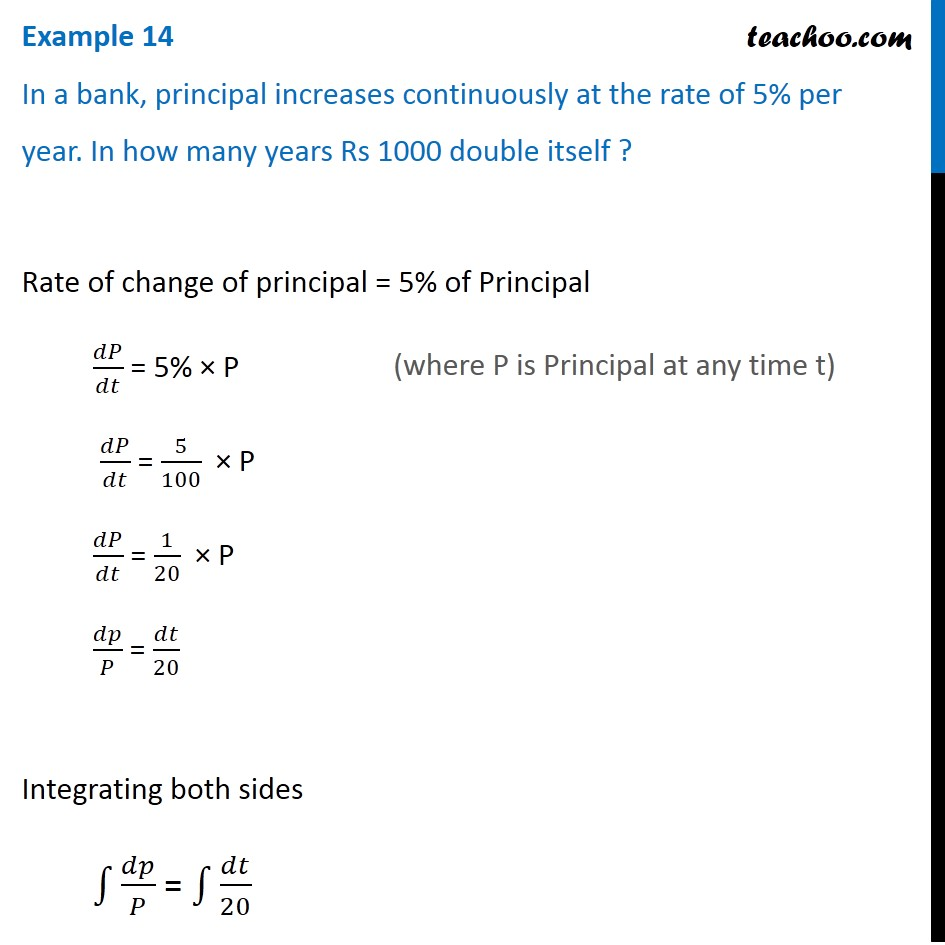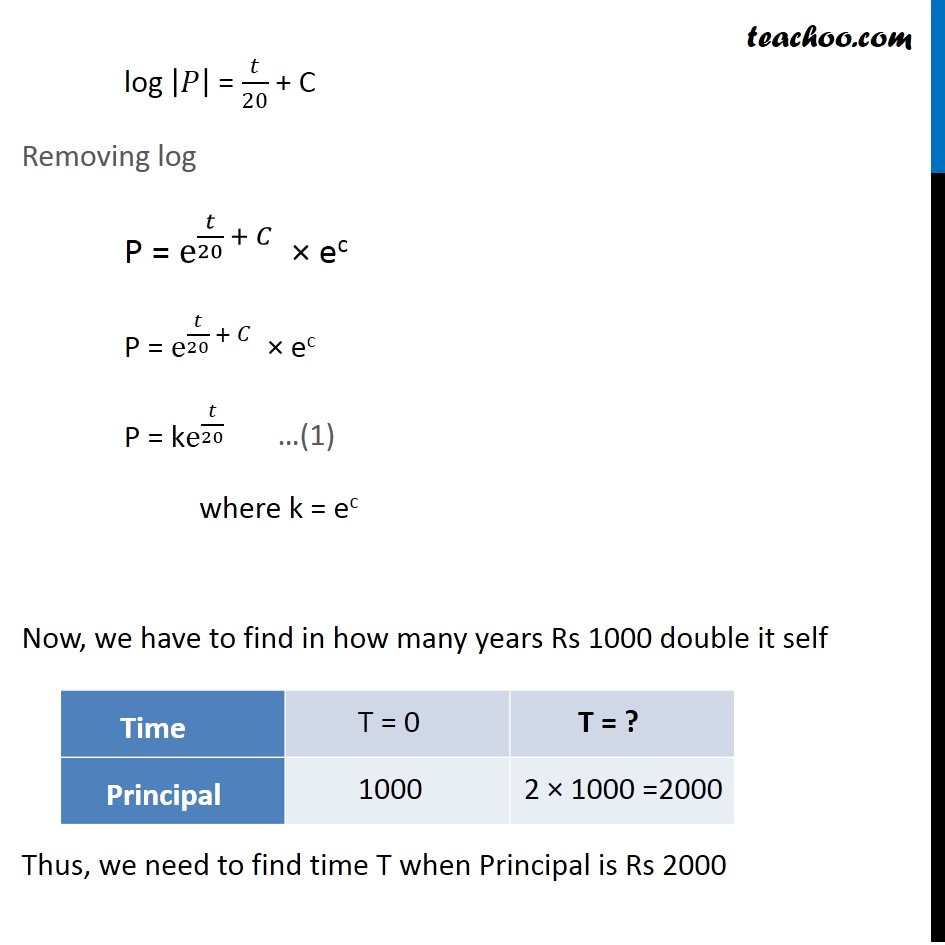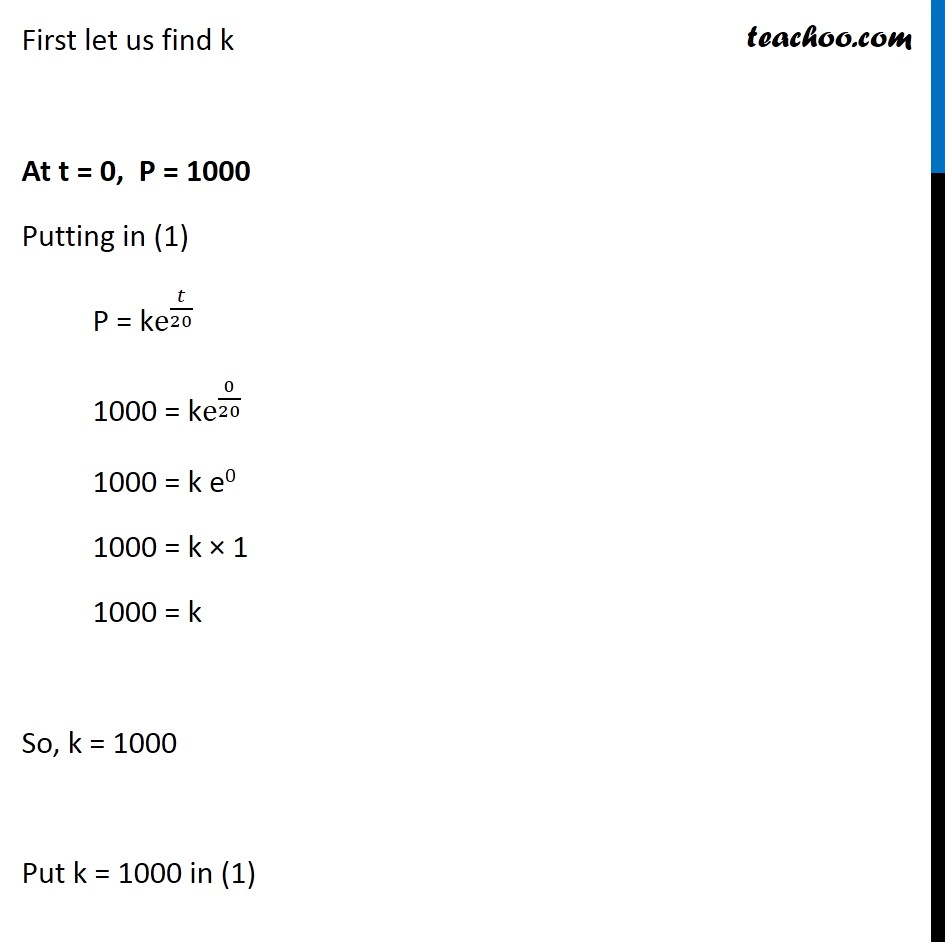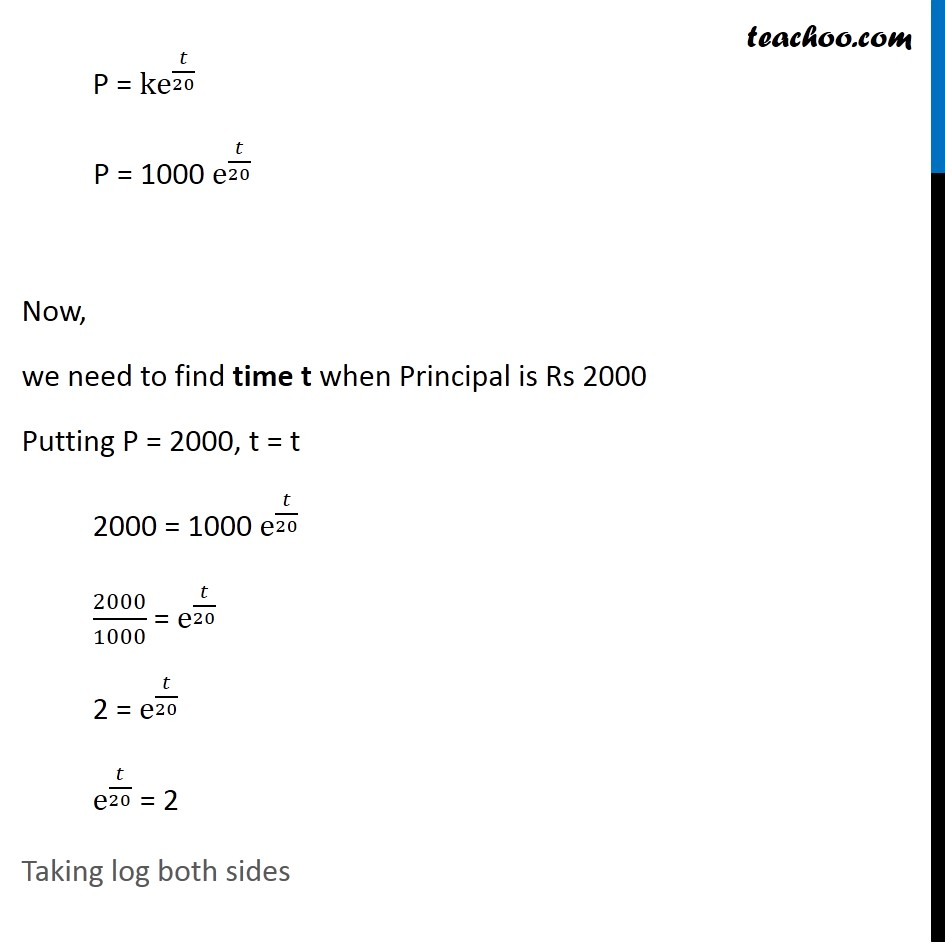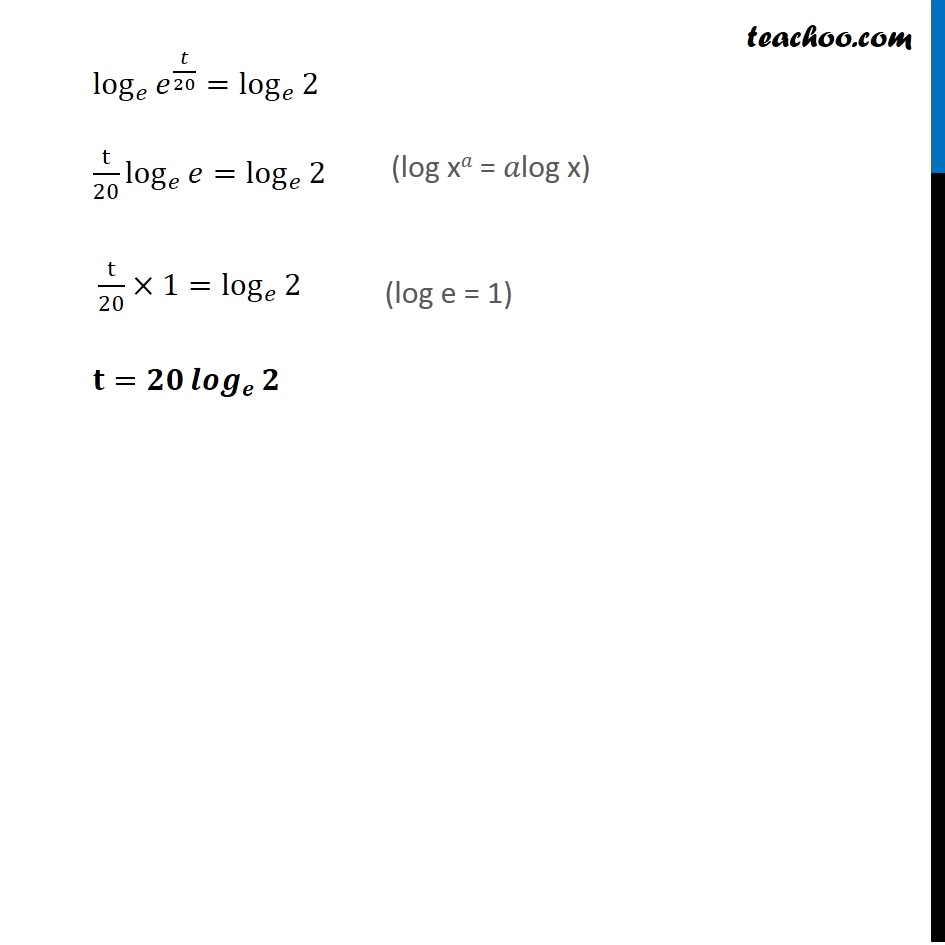Get live Maths 1-on-1 Classs - Class 6 to 12

### Transcript

Example 14 In a bank, principal increases continuously at the rate of 5% per year. In how many years Rs 1000 double itself ? Rate of change of principal = 5% of Principal 𝑑𝑃/𝑑𝑡 = 5% × P 𝑑𝑃/𝑑𝑡 = 5/100 × P 𝑑𝑃/𝑑𝑡 = 1/20 × P 𝑑𝑝/𝑃 = 𝑑𝑡/20 Integrating both sides ∫1▒𝑑𝑝/𝑃 = ∫1▒𝑑𝑡/20 log |𝑃| = 𝑡/20 + C Removing log P = e^(𝑡/20 + 𝐶) × ec P = e^(𝑡/20 + 𝐶) × ec P = ke^(𝑡/20) where k = ec Now, we have to find in how many years Rs 1000 double it self Thus, we need to find time T when Principal is Rs 2000 First let us find k At t = 0, P = 1000 Putting in (1) P = ke^(𝑡/20) 1000 = ke^(0/20) 1000 = k e0 1000 = k × 1 1000 = k So, k = 1000 Put k = 1000 in (1) P = ke^(𝑡/20) P = 1000 e^(𝑡/20) Now, we need to find time t when Principal is Rs 2000 Putting P = 2000, t = t 2000 = 1000 e^(𝑡/20) 2000/1000 = e^(𝑡/20) 2 = e^(𝑡/20) e^(𝑡/20) = 2 Taking log both sides log_𝑒⁡〖𝑒^(𝑡/20) 〗=log_𝑒⁡2 t/20 log_𝑒⁡𝑒=log_𝑒⁡2 " " t/20×1=log_𝑒⁡2 𝐭=𝟐𝟎 〖𝒍𝒐𝒈〗_𝒆⁡𝟐 (log x𝑎 = 𝑎log x) (log e = 1)